# Shortest Path Algorithm

In this tutorial, we will learn the Dijkstra shortest path algorithm but first, let’s briefly introduce some basic concepts of the graph.

First of all, let’s see about the graph.

## What is Graph?

A graph is a representation of a path, a graph helps to understand the paths and shows the directions related to that specified path.

Nodes denote a graph, a node can be any real-life entity and the lines which connect those nodes are called edges which show the relation between them.

There are two types of graphs undirected and directed.

An undirected graph is a type of graph in which we can move in 2-way directions from one node to the other node but if we talk about the directed graph so it is a graph in which we can only move in a defined direction or in a single direction.

The undirected graph connects nodes with lines (edges) and

The directed graph connects the node with arrows like below.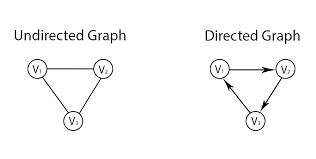Let’s move forward and see what is a weighted graph.

### Weighted graph

It is a graph whose edges contain a number, that number can be anything like cost or weight the number of edges represents the distance or time between two nodes. Have a look at the following image.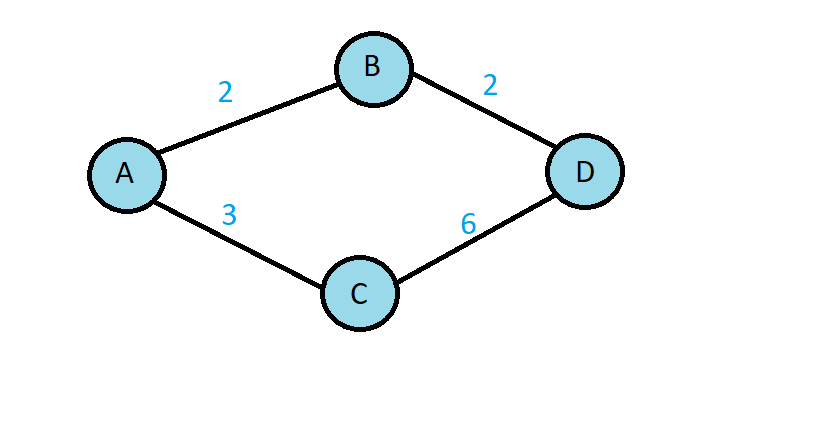I hope you got the concepts now let’s discuss the Dijkstra algorithm.

## Dijkstra algorithm ( shortest path algorithm )

Dijkstra algorithm is called the shortest path algorithm. It is an algorithm that is used to measure or find the shortest path. Map applications like google maps actually use this algorithm.

We used the undirected graph with positive edge values (weights) to find out the shortest path, make sure to use only positive values because we can’t measure the shortest distance with the negative value it will affect the results.

Let’s see how the Dijkstra algorithm works, see the example below.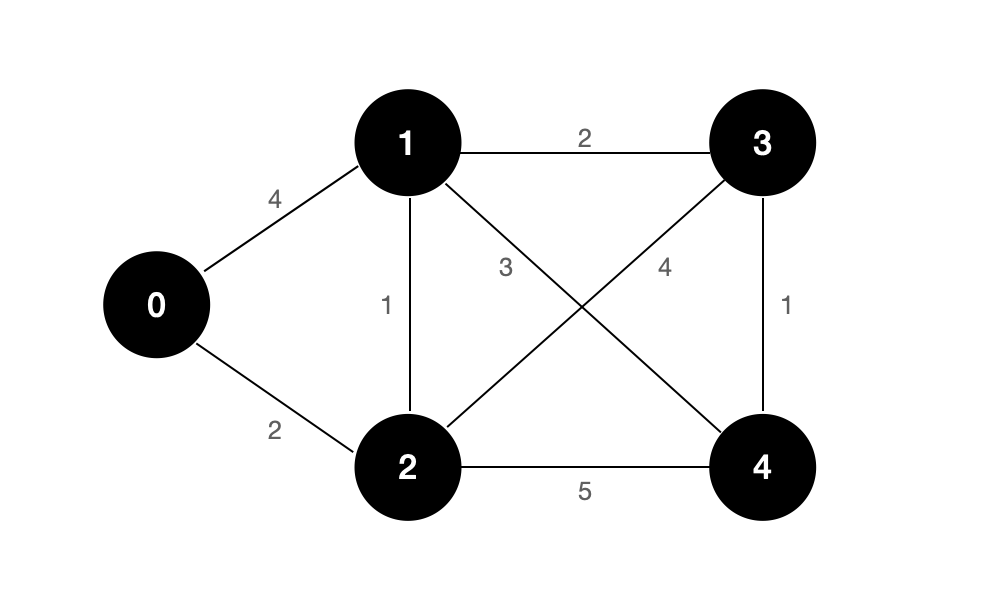First, we select the initial node (source node) from the graph and then start measuring the shortest paths. We will find out the shortest paths throughout all nodes from node0 to node1, node0 to node2 till the end until we visit each node, you can choose any node as a source node we are choosing node0. We did not start finding the shortest paths which means we didn’t visit the nodes yet so the distance between the nodes will be infinite except 0, and the distance of node 0 will remain the same.

Check out the list of nodes below.

As source node 0

0: 0

1: ∞

2: ∞

3: ∞

4: ∞

Let’s start finding the shortest paths.

First, we will check the direct possible edges from the source node that are 0 to 1 and 0 to 2 then we will check the distance between them.

We have to just add the edge value from the source node0 to node 1 which will be 0+4 = 4 as it is, let’s add the node0 and node2 weight which is 2 so it will be like 0+2 =2 our distance list will look like below.

Source node 0

0: 0

1: 4

2: 2

3: ∞

4: ∞

According to our graph, we have visited node0 so we will cut the visited nodes like below.

{ 0,1,2,3,4 }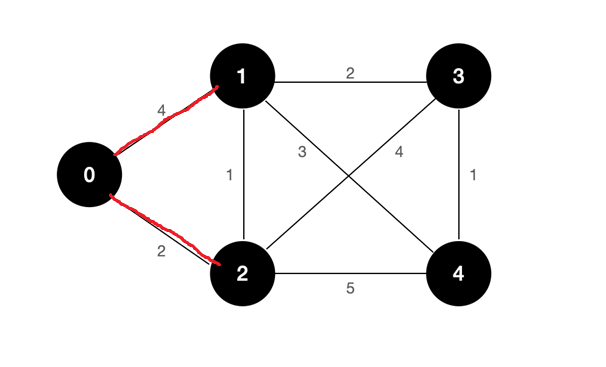The direct path from source node 0 to node1 and node0 to node2 is done, we can’t use zero as a source node now we will select node 1 as the source node. From node1 we have 3 direct paths, node1 to node2, node1 to node3, and node1 to node 4 so we will find the distance between the source node 1 and the direct vertex path node2 first. See the graph below.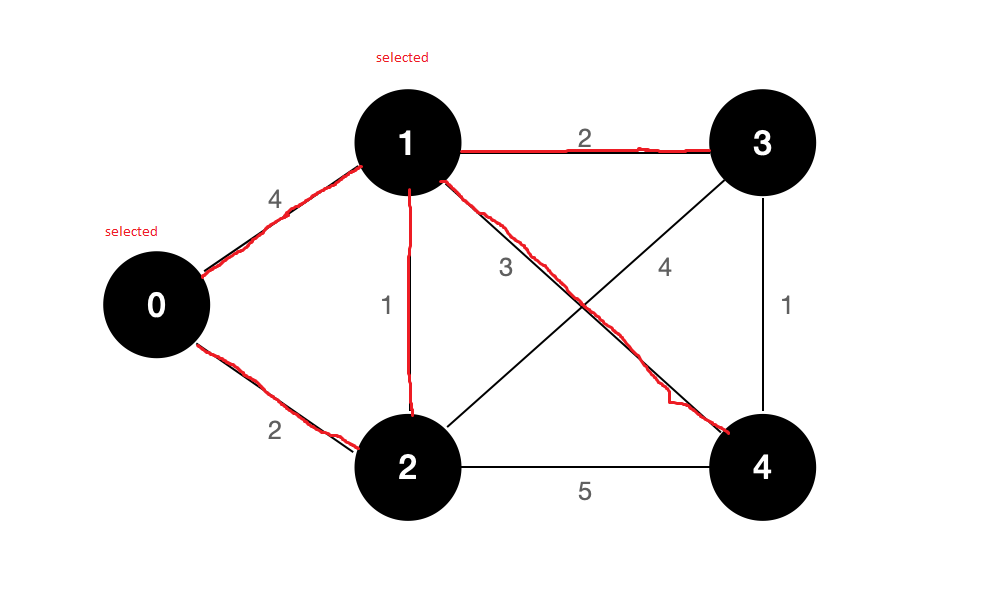we will move from node1 to node2.

As you can see in the graph above the value of node1 is “4” and the distance between node 1 and node 2 is “1” so we will add them 4 +1=5.

Node 1 as a source node

0: minimum cost is 0

1: minimum cost is 4

2: minimum cost is 2 from (1 to 2) is 5

3: from (1 to 3) is 6

4: from (1 to 4) is 7

Visited nodes

{ 0,1,2,3,4 }

Now we will select node 2 as a source node and will the direct shortest paths.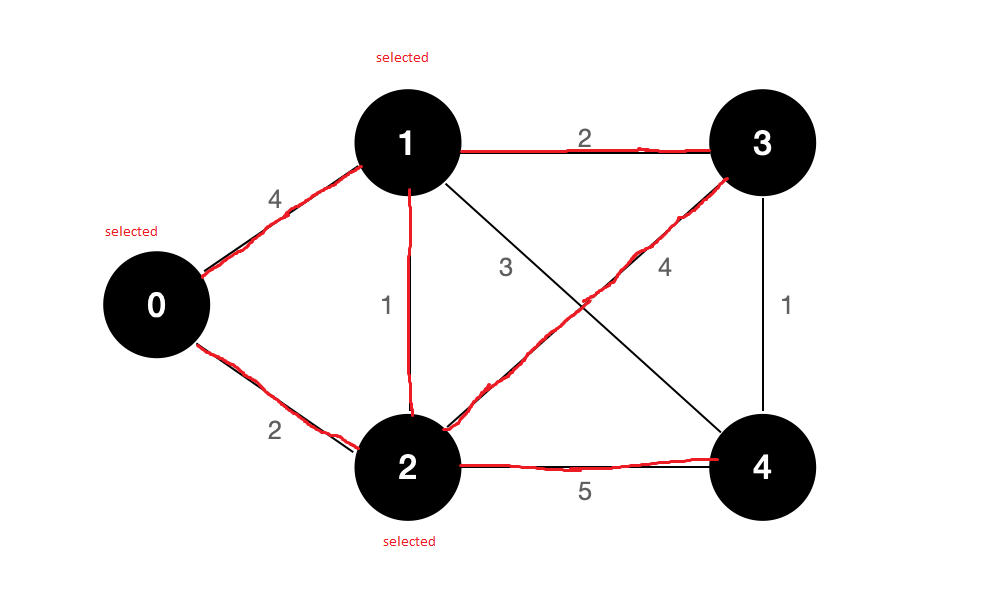As you can see in the above graph there are 2 possible direct paths from 2 to 3 and 2 to 4, we can’t move from 2 to 1 because node1 is already selected we can’t select it again, the value of node 2 is 2 and distance between node2 to node3 is 4 add them 2+4 is “6” as it is, the distance between nod2 to node4 is “5” so we will add them like 4 +5=9.

Node 2 as Source node

0: minimum cost is 0

1: minimum cost is 4

2: minimum cost is 2

3: from (2 to 3) is 6

4: (2 to 4) is 9

Visited nodes

{ 0,1,2,3,4 }

No direct paths are available from node 2 so now we will change the source node from 2 to 3.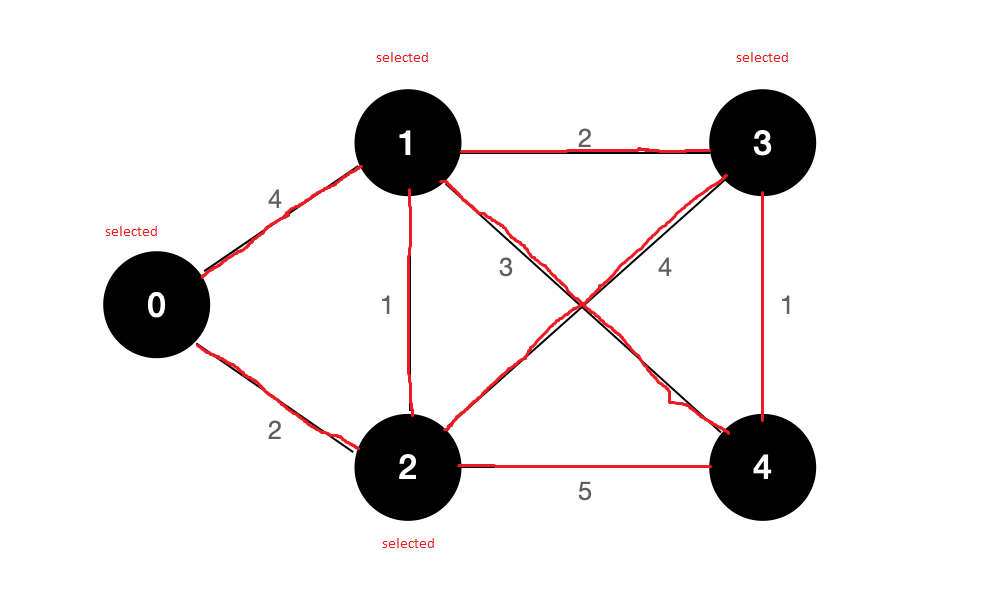Let’s move forward to the second last node as you can see in the above graph the value of node 3 is 2 and the distance between3 to 4 is “1” which will be like 2+1=3. We can’t use the selected nodes for checking the shortest path.

Check out the distance list below:

Node 3 as Source node

0: minimum cost is 0

1: minimum cost is 4

2: minimum cost is 2

3: minimum cost is 2

4: minimum cost is 3

Visited nodes

{ 0,1,2,3,4 }

Now it’s time to move to the last node of the graph, as you can see in the graph below all nodes have been selected and there is no direct node from node 4 so we can’t find the path between node 4 to the other node we will just mark it selected.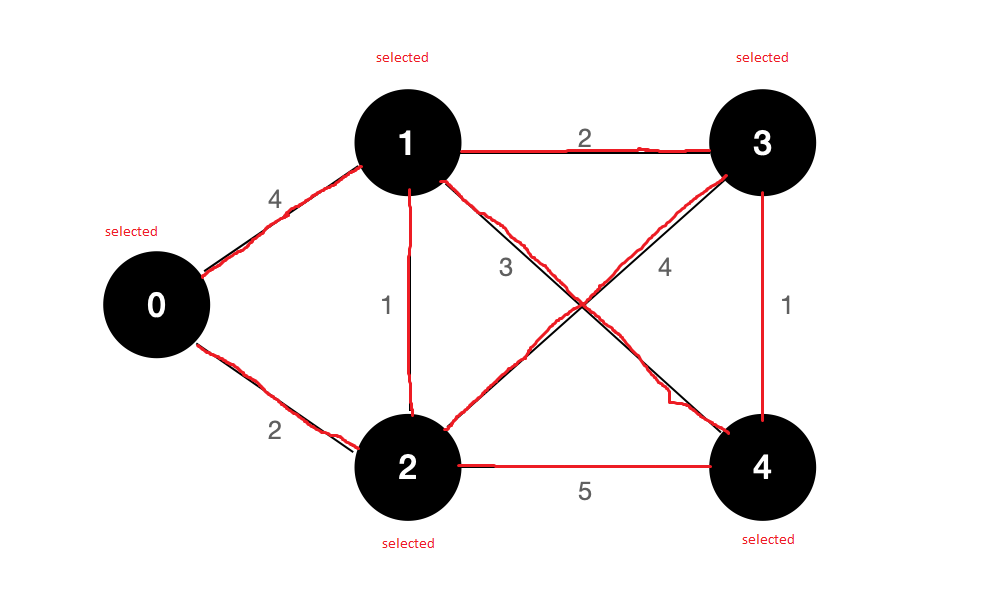Visited nodes

{ 0,1,2,3,4 }

As you can see above our graph has been completed we have found the shortest path using the Dijkstra algorithm.

## Conclusion

In this tutorial, we talked about the shortest path algorithm which is called the Dijkstra algorithm.

We discussed an introduction to the Dijkstra algorithm then we discussed some basic concepts as well related to the algorithm like what is a graph, types of graphs, etc.

We also discussed an example using a graph and applied the Dijkstra algorithm to it to find the shortest path.

That’s all for today hope you like the tutorial if you have any queries regarding this article so feel free to contact us below. Thanks for reading.

Suggested Article: